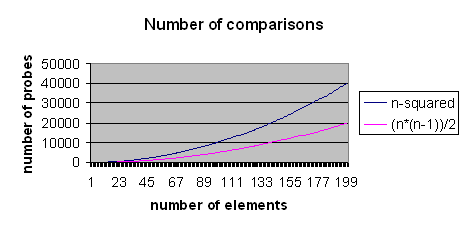## Comparing data structure pairs

Given an array, vector or list of data of any data type there are many situations where it is necessary to do processing on every pair of elements. This document describes and compares such algorithms.

The assumption of this document is that the data is unsorted. It includes C++ and Visual BASIC examples. This may save a student 5 minutes sometime.

### Discussion

Initally I thought that comparing every value with every other value (excluding itself) would be fine, but this is silly. This would compare (4,5) and (5,4) for example which is a waste of time. We only need to compare in one direction.

if we have 0 elements we need 0 comparisons
if we have 1 element we need 0 comparisons

if we have 2 elements we need 1 comparison:
(0,1)
=1

if we have 3 elements we need 3 comparisons:
(0,1), (0,2)
(1,2)
=3

if we have 4 elements we need 6 comparisons:
(0,1), (0,2), (0,3)
(1,2), (1,3)
(2,3)
=6

if we have 5 elements we need 10 comparisons:
(0,1), (0,2), (0,3), (0,4)
(1,2), (1,3), (1,4)
(2,3), (2,4)
(3,4)
=10

if we have 6 elements we need 15 comparisons:
(0,1), (0,2), (0,3), (0,4), (0,5)
(1,2), (1,3), (1,4), (1,5)
(2,3), (2,4), (2,5)
(3,4), (3,5)
(4,5)
=15

### Algorithm

From these examples we can see a pattern emerge that in the comparison (n,m) m is always at least one greater than n so we only need to compare half the number of elements excluding itself.

compare every element with every other element requires n*n probes,
half the number of elements to give us (n*n)/2,
exclude itself to give (n*(n-1))/2

### ComparisonThis graph compares n-squared with (n*(n-1))/2. This algorithm requires far fewer probes and is therefore far more efficient.

### Code examples

#### C++

 `````` // mincompares: (n*(n-1))/2 void mincompares(int n) { int x,y; unsigned long nc=0; for(x=0;x

#### Visual BASIC

 `````` Private Sub mincompares(lngProbes As Long) Dim n As Long Dim m As Long Dim lngProbesM As Long Dim ct As Long lngProbesM = lngProbes - 1 For n = 0 To lngProbesM For m = n + 1 To lngProbesM ' do something useful, compare(ob(n),ob(m)) ct = ct + 1 Next m Next n MsgBox Trim\$(Str\$(ct)) End Sub ``````

### Summary

If the data is sorted other order log2(n) algorithms can be used. For string data hash tables can provide even more efficiency to quickly identify matches but for some situations for ease of development this algorithm is fine.

Back to index.